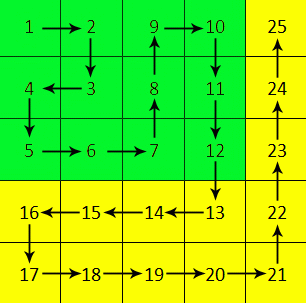### Problem Statement

Let us define an infinite table of integers as follows:Each cell is uniquely identified by the value it contains. Let us define S(X) as the sum of all the values in the rectangle with cell 1 as its upper-left corner and cell X as its lower-right corner. For example, S(12) is the sum of all the values in the green rectangle shown above.

You are given a long N. First, find the rectangle with cell 1 as its upper-left corner and cell N as its lower-right corner. Then, calculate the sum of S(X) for all values X inside this rectangle. Return this sum modulo 1,000,000,007.

For example, if N is 8, you would first find the 3 x 2 rectangle with 1 in its upper-left corner and 8 in its lower-right corner. You would then calculate S(X) for each value X in this rectangle: S(1) = 1, S(2) = 3, S(9) = 12, S(4) = 5, S(3) = 10, and S(8) = 27. You would them sum these values to get 1 + 3 + 12 + 5 + 10 + 27 = 58.

### Definition

 Class: MegaSum Method: calculate Parameters: long Returns: int Method signature: int calculate(long N) (be sure your method is public)

### Constraints

-N will be between 1 and 10^10, inclusive.

### Examples

0)

 `8`
`Returns: 58`
 This is the example from the problem statement.
1)

 `12`
`Returns: 282`
2)

 `11`
`Returns: 128`
3)

 `6`
`Returns: 50`
4)

 `34539`
`Returns: 437909839`

#### Problem url:

http://www.topcoder.com/stat?c=problem_statement&pm=10710

#### Problem stats url:

http://www.topcoder.com/tc?module=ProblemDetail&rd=13908&pm=10710

Gluk

#### Testers:

PabloGilberto , kalinov , ivan_metelsky

Math